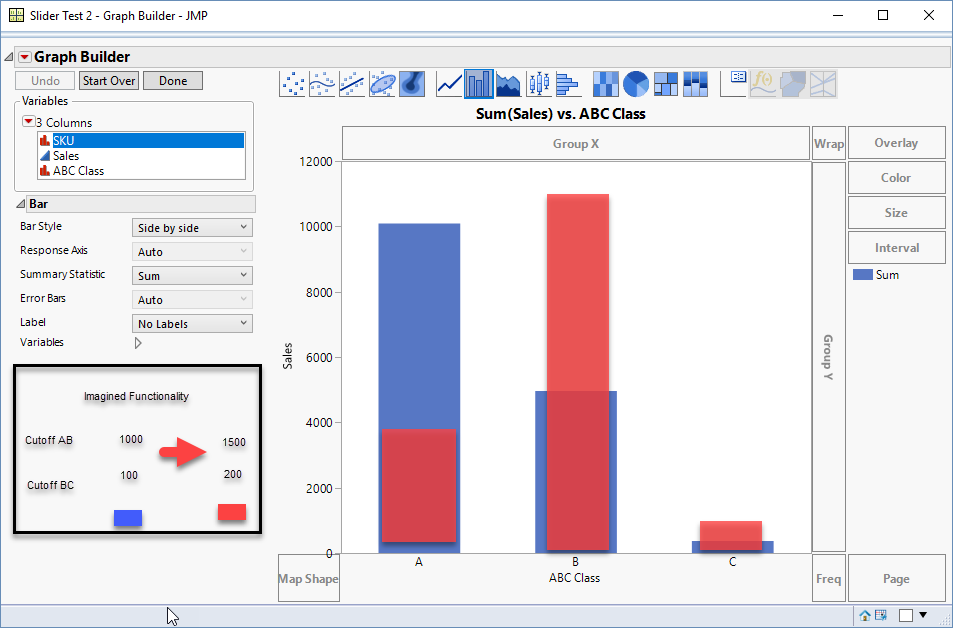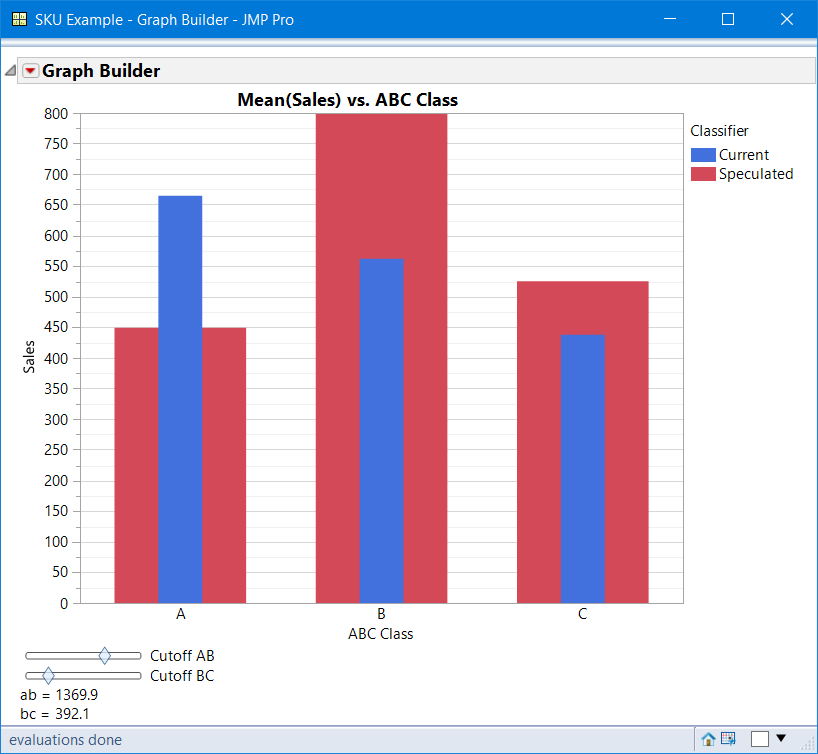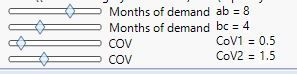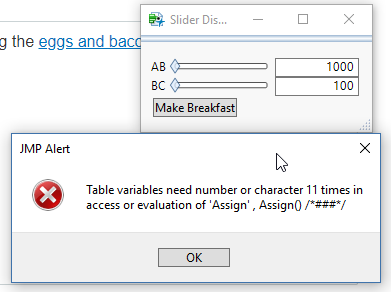Our World Statistics Day conversations have been a great reminder of how much statistics can inform our lives. Do you have an example of how statistics has made a difference in your life? Share your story with the Community!
Choose Language Hide Translation Bar
Highlighted

## How to have adjustable variables available in the graph so the graph adjusts in realtime? (recalcuate, interactive, sensitivity analysis)

I'm not sure what to call it.  Maybe table variables can do this. Maybe predition profiler can.

In the end I would want a formula column ABC Class that is a result of at least two of what I'll call "global parameters".  Basic ABC SKU classification sets cutoffs as the aggregate sum of all the SKUs set revenue %s.  As should be 80% of revenue, Bs 15% and Cs 5%.  For this example I'll make it even simpler, let's say the SKU gets classified as A, B or C by the sales on that SKU.

In the example below,  there are three rules (which I did not code as a formula),set by two parameters.

• SKUs with sales of more than 1000 -> A
• SKUs with sales of more than 100 -> B
• The rest ->C

Example datatable

``````//create datatable
dt = New Table( "Slider Test",
New Column( "SKU",
Character,
"Nominal",
Set Selected,
Set Values(
{"A", "B", "C", "D", "E", "F", "G", "H", "I", "J", "K", "L", "M", "N",
"O", "P", "Q", "R", "S", "T", "U", "V", "W", "X", "Y", "Z"}
)
),
New Column( "Sales",
Numeric,
"Continuous",
Format( "Best", 12 ),
Set Values(
[98, 64, 120, 397, 93, 58, 196, 700, 674, 229, 607, 55, 590, 169, 1449,
1236, 143, 1714, 364, 404, 226, 1300, 1105, 144, 1391, 1893]
),
Set Display Width( 94 )
),
New Column( "ABC Class",
Character,
"Nominal",
Set Values(
{"C", "C", "B", "B", "C", "C", "B", "B", "B", "B", "B", "C", "B", "B",
"A", "A", "B", "A", "B", "B", "B", "A", "A", "B", "A", "A"}
),
Set Display Width( 70 )
)
);

//create graph
dt <<Graph Builder(
Variables( X( :ABC Class ), Y( :Sales ) ),
Elements( Bar( X, Y, Legend( 6 ), Summary Statistic( "Sum" ) ) )
)``````

Example image.  It would be great if I could type or have a slider for the two variables shown.

In the example below,  there are three rules (which I did not code as a formula),set by two parameters (cutoff AB and cutoff BC).

• SKUs with sales of more than 1000 -> A             then I show the result changing it to 1500
• SKUs with sales of more than 100 -> B                then I show the result chaning it to 150
• The rest ->CThank you.

2 ACCEPTED SOLUTIONS

Accepted Solutions
Highlighted

## Re: How to have adjustable variables available in the graph so the graph adjusts in realtime? (recalcuate, interactive, sensitivity analysis)

@BSwid, your graph using GraphBuilder is a complex because there are so many dynamic components, and what is the best method depends upon usage.  For example, if this graph is to be used by others, they could change the  GraphBuider Summary Statistic for Sales to be the Max or MIn o Std Dev instead of the Mean.  In other words, there is the added complexity of maintaining the features of GraphBuilder.

If this summary statistic was not dynamic, this could be achieved by adding a custom function to the framebox of the GB. It would require computing the mean for each cutoff change. and redrawing the graph. However, if rows were excluded, you would need to script a row state handler so your special bars would be computed. Also, another column can be chosen for X-Group , etc., then the dynamic slider interface could have problems.

When using a JMP platform, I prefer to use the features of JMP even it if requires restructuring the table to do so.  For GB to draw these nested bars, there needs to be a variable to specify the current bars and then the speculated bars, which would be used as an Overlay, and the bars can be side-by-side or nested. From your drawing, I chose nested, but with thiis script you can change that,

To restructure your data, you need 2 copies, the original and the speculated values. The method I chose was to concatenate the table with itself. This script has an assumption, that the current classification is from a script, if not the formula defined would need to be modified and likley require a new column.Attached are 3 files:

• original table, SKU Example.jmp
• modified table SKU Example_Final.jmp Note: The script to create the interactive GB is attached to this table
• script, SKU script.jsl opens the original table from c:\temp. you will need to modifiy to your path.  This script restructures the tabel abd draws the dynamic graph.

Note: it might be easier to just create a dynamic custom graph, that has a fixed summary statistic and no other bells and whistles to maintain. This method was used to document the issues with scripting a custom dynamic widget with GraphBuilder.

Good Luck.

Highlighted

## Re: How to have adjustable variables available in the graph so the graph adjusts in realtime? (recalcuate, interactive, sensitivity analysis)

The example was a tremendous help. I was able to achieve exactly what I imagined with your help. Thank you.

Here's what I eneded up doing to get multiple sliders and have the global box to the right of the slider.

``````//graph builder code above here (not shown)
//code for slider below

//number of months of demand variables
ab = dt:Cutoff AB;
bc = dt:Cutoff BC;
//CoV variables
CoV1 = dt:CoV1;
CoV2 = dt:CoV2;

:ABC Class << Suppress Eval( 0 );

specBox = H List Box(
V List Box(
//number of months of demand
H List Box(
Slider Box(
0,
12,
ab,
dt:Cutoff AB = ab;
dt << rerun formulas;
),
Text Box( "Months of demand" )
),
H List Box(
Slider Box(
0,
12,
bc,
dt:Cutoff BC = bc;
dt << rerun formulas;
),
Text Box( "Months of demand" )
),
//CoV
H List Box(
Slider Box(
0,
4,
CoV1,
dt:CoV1 = CoV1;
dt << rerun formulas;
),
Text Box( "COV" )
),
H List Box(
Slider Box(
0,
4,
CoV2,
dt:CoV2 = CoV2;
dt << rerun formulas;
),
Text Box( "COV" )
),
),//end V list box 1

//display the variables
V List Box(
Global Box( ab ),
Global Box( bc ),
Global Box( CoV1 ),
Global Box( CoV2 )
)//end V list box2
);//end H list box
(Report( gb )[Picture Box( 1 )] << Parent) << Append( specBox );``````6 REPLIES 6
Highlighted

## Re: How to have adjustable variables available in the graph so the graph adjusts in realtime? (recalcuate, interactive, sensitivity analysis)

I tried setting table variables :AB and :BC. and then tried modifying the eggs and bacon example from the jsl cookbook.

But when I try to move the slider I get an error.``````//use table variables instead :AB & :BC
//Eggs = 50;      // :AB
//Bacon = 50;     // :BC

//window
New Window( "Slider Display",
Border Box( Left( 10 ), Right( 10 ), top( 10 ), bottom( 10 ),
V List Box(
H List Box(
Text Box( "AB" ), // slider label
ABsb = Slider Box( 0, 2000, :AB, // slider script follows...
ABnb << set( :AB ); // update the value
// this example doesn't need any other interactive
// update. You could do some other work here, using
// the slider's value to change a graph, perhaps.
),
// text box to hold the slider's value...
ABnb = Number Edit Box( :AB, <<setfunction(
Function( {this}, // if the text box value changes
:AB = this << get; // get the value
ABsb << inval; // update the slider
)
)
)
),
H List Box(
Text Box( "BC" ), // slider label
BCsb = Slider Box( 0, 100, :BC, // slider script follows...
BCnb << set( :BC ); // update the value
),
// text box to hold the slider's value...
BCnb = Number Edit Box( :BC, <<setfunction(
Function( {this}, // if the text box value changes
:BC = this << get; // get the value
BCsb << inval; // update the slider
)
)
)
),
Button Box( "Make Breakfast", // button script follows...
New Window( "Breakfast", Text Box( "eggs: " || Char( :AB ) || " and bacon: " || Char( :BC ) ) )
)
)
)
);``````

(I think ) I want the slider box to update the table variable, which updates the formula in the data table and thus the graph.

I'm certain I'll need this line.

``gb<<inval; // update the graphbox``
Highlighted

## Re: How to have adjustable variables available in the graph so the graph adjusts in realtime? (recalcuate, interactive, sensitivity analysis)

@BSwid wrote:

I'm not sure what to call it.  Maybe table variables can do this. Maybe predition profiler can.

In the end I would want a formula column ABC Class that is a result of at least two of what I'll call "global parameters".  Basic ABC SKU classification sets cutoffs as the aggregate sum of all the SKUs set revenue %s.  As should be 80% of revenue, Bs 15% and Cs 5%.  For this example I'll make it even simpler, let's say the SKU gets classified as A, B or C by the sales on that SKU.

In the example below,  there are three rules (which I did not code as a formula),set by two parameters.

• SKUs with sales of more than 1000 -> A
• SKUs with sales of more than 100 -> B
• The rest ->C

Example datatable

``````//create datatable
dt = New Table( "Slider Test",
New Column( "SKU",
Character,
"Nominal",
Set Selected,
Set Values(
{"A", "B", "C", "D", "E", "F", "G", "H", "I", "J", "K", "L", "M", "N",
"O", "P", "Q", "R", "S", "T", "U", "V", "W", "X", "Y", "Z"}
)
),
New Column( "Sales",
Numeric,
"Continuous",
Format( "Best", 12 ),
Set Values(
[98, 64, 120, 397, 93, 58, 196, 700, 674, 229, 607, 55, 590, 169, 1449,
1236, 143, 1714, 364, 404, 226, 1300, 1105, 144, 1391, 1893]
),
Set Display Width( 94 )
),
New Column( "ABC Class",
Character,
"Nominal",
Set Values(
{"C", "C", "B", "B", "C", "C", "B", "B", "B", "B", "B", "C", "B", "B",
"A", "A", "B", "A", "B", "B", "B", "A", "A", "B", "A", "A"}
),
Set Display Width( 70 )
)
);

//create graph
dt <<Graph Builder(
Variables( X( :ABC Class ), Y( :Sales ) ),
Elements( Bar( X, Y, Legend( 6 ), Summary Statistic( "Sum" ) ) )
)``````

Example image.  It would be great if I could type or have a slider for the two variables shown.

In the example below,  there are three rules (which I did not code as a formula),set by two parameters (cutoff AB and cutoff BC).

• SKUs with sales of more than 1000 -> A             then I show the result changing it to 1500
• SKUs with sales of more than 100 -> B                then I show the result chaning it to 150
• The rest ->CThank you.

I tried setting table variables :AB and :BC. and then tried modifying the eggs and bacon example from the jsl cookbook.

But when I try to move the slider I get an error.``````//use table variables instead :AB & :BC
//Eggs = 50;      // :AB
//Bacon = 50;     // :BC

//window
New Window( "Slider Display",
Border Box( Left( 10 ), Right( 10 ), top( 10 ), bottom( 10 ),
V List Box(
H List Box(
Text Box( "AB" ), // slider label
ABsb = Slider Box( 0, 2000, :AB, // slider script follows...
ABnb << set( :AB ); // update the value
// this example doesn't need any other interactive
// update. You could do some other work here, using
// the slider's value to change a graph, perhaps.
),
// text box to hold the slider's value...
ABnb = Number Edit Box( :AB, <<setfunction(
Function( {this}, // if the text box value changes
:AB = this << get; // get the value
ABsb << inval; // update the slider
)
)
)
),
H List Box(
Text Box( "BC" ), // slider label
BCsb = Slider Box( 0, 100, :BC, // slider script follows...
BCnb << set( :BC ); // update the value
),
// text box to hold the slider's value...
BCnb = Number Edit Box( :BC, <<setfunction(
Function( {this}, // if the text box value changes
:BC = this << get; // get the value
BCsb << inval; // update the slider
)
)
)
),
Button Box( "Make Breakfast", // button script follows...
New Window( "Breakfast", Text Box( "eggs: " || Char( :AB ) || " and bacon: " || Char( :BC ) ) )
)
)
)
);``````

(I think ) I want the slider box to update the table variable, which updates the formula in the data table and thus the graph.

I'm certain I'll need this line.

``gb<<inval; // update the graphbox``

Highlighted

## Re: How to have adjustable variables available in the graph so the graph adjusts in realtime? (recalcuate, interactive, sensitivity analysis)

@BSwid, your graph using GraphBuilder is a complex because there are so many dynamic components, and what is the best method depends upon usage.  For example, if this graph is to be used by others, they could change the  GraphBuider Summary Statistic for Sales to be the Max or MIn o Std Dev instead of the Mean.  In other words, there is the added complexity of maintaining the features of GraphBuilder.

If this summary statistic was not dynamic, this could be achieved by adding a custom function to the framebox of the GB. It would require computing the mean for each cutoff change. and redrawing the graph. However, if rows were excluded, you would need to script a row state handler so your special bars would be computed. Also, another column can be chosen for X-Group , etc., then the dynamic slider interface could have problems.

When using a JMP platform, I prefer to use the features of JMP even it if requires restructuring the table to do so.  For GB to draw these nested bars, there needs to be a variable to specify the current bars and then the speculated bars, which would be used as an Overlay, and the bars can be side-by-side or nested. From your drawing, I chose nested, but with thiis script you can change that,

To restructure your data, you need 2 copies, the original and the speculated values. The method I chose was to concatenate the table with itself. This script has an assumption, that the current classification is from a script, if not the formula defined would need to be modified and likley require a new column.Attached are 3 files:

• original table, SKU Example.jmp
• modified table SKU Example_Final.jmp Note: The script to create the interactive GB is attached to this table
• script, SKU script.jsl opens the original table from c:\temp. you will need to modifiy to your path.  This script restructures the tabel abd draws the dynamic graph.

Note: it might be easier to just create a dynamic custom graph, that has a fixed summary statistic and no other bells and whistles to maintain. This method was used to document the issues with scripting a custom dynamic widget with GraphBuilder.

Good Luck.

Highlighted

## Re: How to have adjustable variables available in the graph so the graph adjusts in realtime? (recalcuate, interactive, sensitivity analysis)

@gzmorgan0 Thank you I'll review the examples.
Based on reading the response, I should clarify I wasn't trying to show the current and speculated at the same time, just simply to have the graph (summary statistic) update as the parameters AB & BC change.
Highlighted

## Re: How to have adjustable variables available in the graph so the graph adjusts in realtime? (recalcuate, interactive, sensitivity analysis)

If you do not want a comparison of two different classifiers, then all you need is:

1. a formula for column :ABC Class that uses the two table variable Cutoff AB and Cutoff BC
2. The GraphBuilder script without the overlay variable :Classifier

No need to restructure the table (concatenate etc.)  This is a much simpler problem.

Highlighted

## Re: How to have adjustable variables available in the graph so the graph adjusts in realtime? (recalcuate, interactive, sensitivity analysis)

The example was a tremendous help. I was able to achieve exactly what I imagined with your help. Thank you.

Here's what I eneded up doing to get multiple sliders and have the global box to the right of the slider.

``````//graph builder code above here (not shown)
//code for slider below

//number of months of demand variables
ab = dt:Cutoff AB;
bc = dt:Cutoff BC;
//CoV variables
CoV1 = dt:CoV1;
CoV2 = dt:CoV2;

:ABC Class << Suppress Eval( 0 );

specBox = H List Box(
V List Box(
//number of months of demand
H List Box(
Slider Box(
0,
12,
ab,
dt:Cutoff AB = ab;
dt << rerun formulas;
),
Text Box( "Months of demand" )
),
H List Box(
Slider Box(
0,
12,
bc,
dt:Cutoff BC = bc;
dt << rerun formulas;
),
Text Box( "Months of demand" )
),
//CoV
H List Box(
Slider Box(
0,
4,
CoV1,
dt:CoV1 = CoV1;
dt << rerun formulas;
),
Text Box( "COV" )
),
H List Box(
Slider Box(
0,
4,
CoV2,
dt:CoV2 = CoV2;
dt << rerun formulas;
),
Text Box( "COV" )
),
),//end V list box 1

//display the variables
V List Box(
Global Box( ab ),
Global Box( bc ),
Global Box( CoV1 ),
Global Box( CoV2 )
)//end V list box2
);//end H list box
(Report( gb )[Picture Box( 1 )] << Parent) << Append( specBox );``````Article Labels

There are no labels assigned to this post.